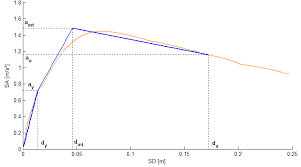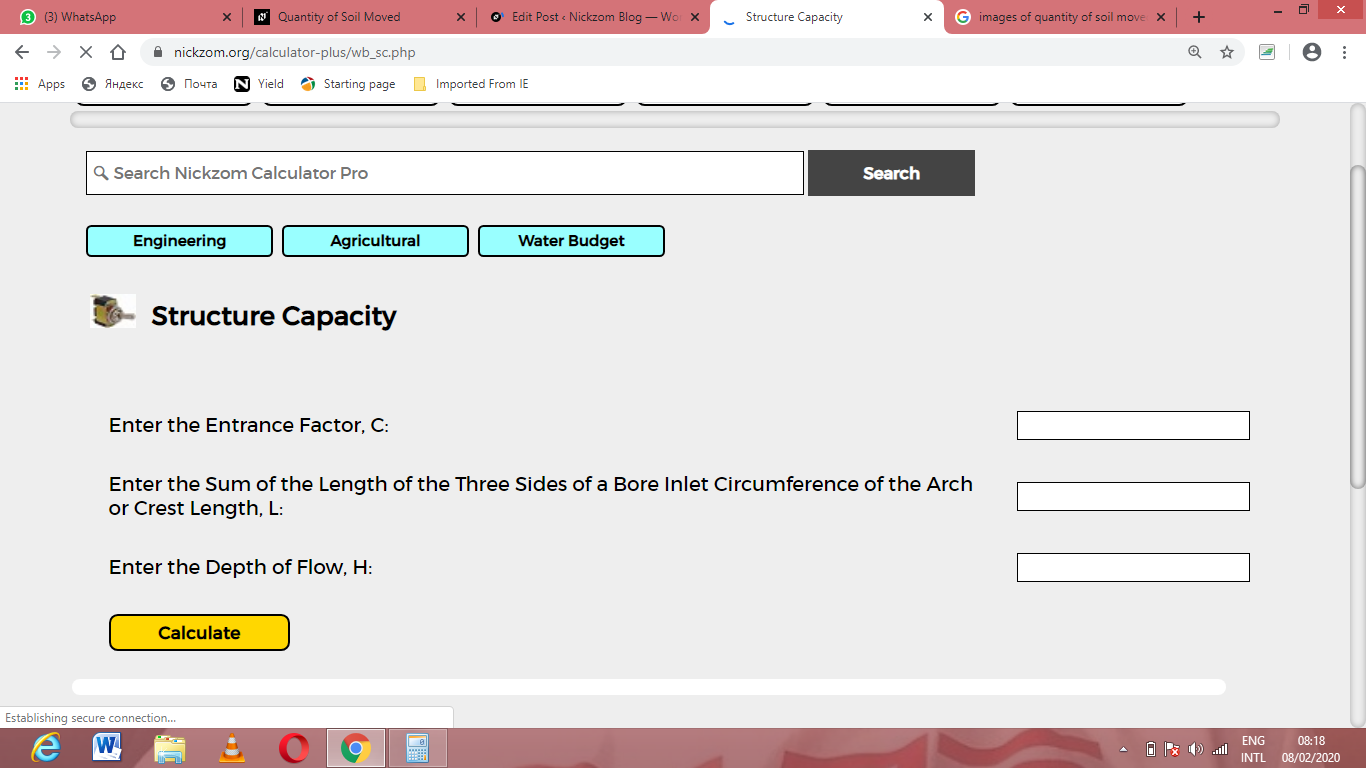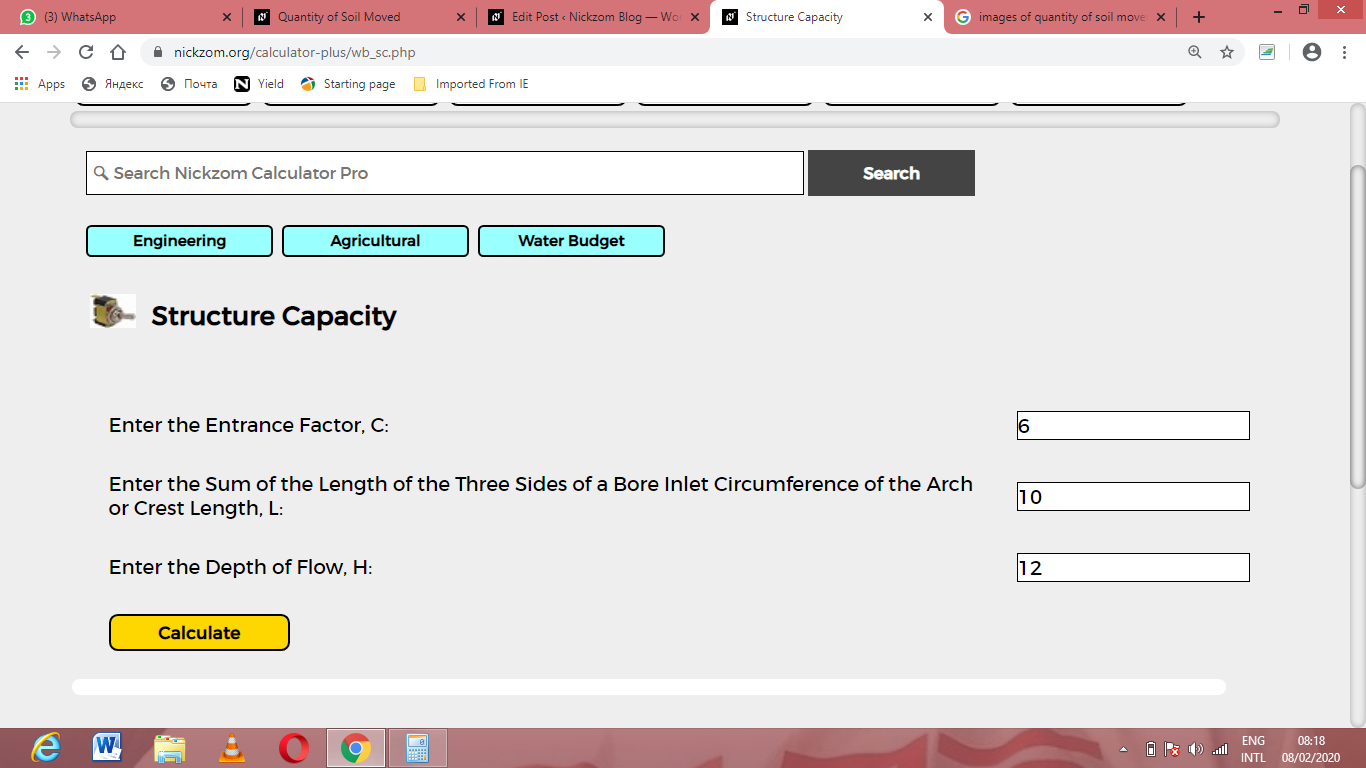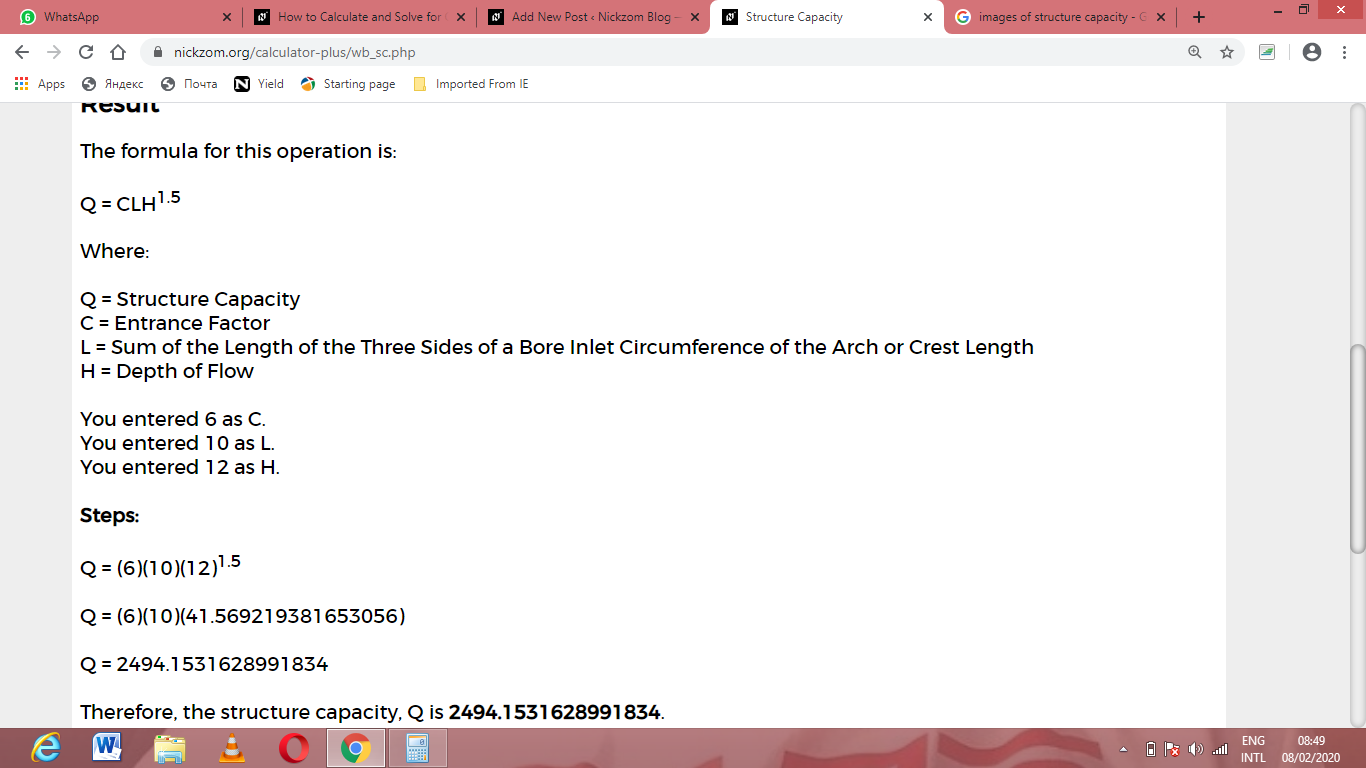# How to Calculate and Solve for Structure Capacity | Water BudgetThe image above represents structure capacity.

To compute for structure capacity, three essential parameters are needed and these parameters are Entrance Factor (C), Crest Length (L) and Depth of Flow (H).

The formula for calculating the structure capacity:

Q = CLH1.5

Where:

Q = Structure Capacity
C = Entrance Factor
L = Crest Length
H = Depth of Flow

Let’s solve an example;
Find the structure capacity when the entrance factor is 6, the crest length is 10 and the depth of flow is 12.

This implies that;

C = Entrance Factor = 6
L = Crest Length = 10
H = Depth of Flow = 12

Q = CLH1.5
Q = (6)(10)(12)1.5
Q = (6)(10)(41.56)
Q = 2494.15

Therefore, the structure capacity is 2494.15.

Calculating the Entrance Factor when the Structure Capacity, the Crest Length and the Depth of Flow is Given.

C = Q / LH1.5

Where;

C = Entrance Factor
Q = Structure Capacity
L = Crest Length
H = Depth of Flow

Let’s solve an example;
Find the entrance factor when the structure capacity is 20, the crest length is 8 and the depth of flow is 4.

This implies that;

Q = Structure Capacity = 20
L = Crest Length = 8
H = Depth of Flow = 4

C = Q / LH1.5
C = 20 / (8)(8)
C = 20 / 64
C = 0.3125

Therefore, the entrance factor is 0.3125.

Calculating the Crest Length when the Structure Capacity, the Entrance Factor and the Depth of Flow is Given.

L = Q / CH1.5

Where;

L = Crest Length
C = Entrance Factor
Q = Structure Capacity
H = Depth of Flow

Let’s solve an example;
Find the crest length when the entrance factor is 10, the structure capacity is 30 and the depth of flow is 2.

This implies that;

C = Entrance Factor = 10
Q = Structure Capacity = 30
H = Depth of Flow = 2

L = Q / CH1.5
L = 30 / (10)(21.5)
L = 30 / (10)(2.82)
L = 30 / 28.2
L = 1.06

Therefore, the crest length is 1.06.

Calculating the Depth of Flow when the Structure Capacity, the Entrance Factor and the Crest Length is Given.

H = 1.5Q / CL

Where;

H = Depth of Flow
L = Crest Length
C = Entrance Factor
Q = Structure Capacity

Let’s solve an example;
Find the depth of flow when the structure capacity is 24, the entrance factor is 12 and the crest length is 10.

This implies that;

L = Crest Length = 10
C = Entrance Factor = 12
Q = Structure Capacity = 24

H = 1.5Q / CL
H = 1.524 / (12)(10)
H = 1.524 / 120
H = 1.5√0.2
H = 0.34

Therefore, the depth of flow is 0.34.

Nickzom Calculator – The Calculator Encyclopedia is capable of calculating the structure capacity.

To get the answer and workings of the structure capacity using the Nickzom Calculator – The Calculator Encyclopedia. First, you need to obtain the app.

You can get this app via any of these means:

To get access to the professional version via web, you need to register and subscribe for NGN 1,500 per annum to have utter access to all functionalities.
You can also try the demo version via https://www.nickzom.org/calculator

Apple (Paid) – https://itunes.apple.com/us/app/nickzom-calculator/id1331162702?mt=8
Once, you have obtained the calculator encyclopedia app, proceed to the Calculator Map, then click on Agricultural under Engineering.Now, Click on Water Budget under AgriculturalNow, Click on Structure Capacity under Water BudgetThe screenshot below displays the page or activity to enter your values, to get the answer for the structure capacity according to the respective parameters which are the Entrance Factor (C), Crest Length (L) and Depth of Flow (H).Now, enter the values appropriately and accordingly for the parameters as required by the Entrance Factor (C) is 6, Crest Length (L) is 10 and Depth of Flow (H) is 12.Finally, Click on CalculateAs you can see from the screenshot above, Nickzom Calculator– The Calculator Encyclopedia solves for the structure capacity and presents the formula, workings and steps too.